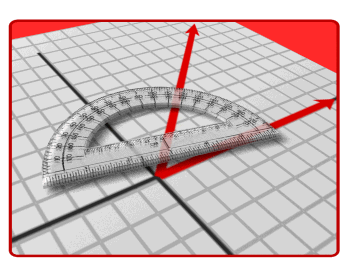•Math Program - EnVisions Overview

Chapter 1: Numeration

Chapter 2: Variables, Expressions, Properties

Chapter 3: Operations with Decimals

Chapter 4: Solving Equations

Chapter 5: Number and Fraction Concepts

Chapter 6: Decimals, Fractions, Mixed Numbers

Chapter 7: Adding & Subtracting Fractions and Mixed Numbers

Chapter 8: Multiplying Fractions and Mixed Numbers

Chapter 9: Dividing Fractions and Mixed Numbers

Chapter 10: Integers

Chapter 11: Properties of 2 Dimensional Figures

Chapter 12: Ratios, Rates, and Proportions

Chapter 13: Solving Proportions

Chapter 14: Understanding Percent

Chapter 15: Equations and Graphs

Chapter 16: Measurement

Chapter 17: Perimeter and Area

Chapter 18: Volume and Surface Area

Chapter 19: Data, Statistics, and Graphs